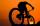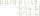The hiker

The hiker will travel 40% of the route on the first day 1and/3 of the rest od second day. Last day 30 km. What was the length of the 3-day trip? How many kilometers did he walk each day?

Result

a =  30
b =  15
c =  30
t =  75

Solution:

a+b+c=t
a = 40/100t
b = 1/3*(t-a)
c = 30

a+b+c-t = 0
100a-40t = 0
a+3b-t = 0
c = 30

a = 30
b = 15
c = 30
t = 75

Calculated by our linear equations calculator.

Leave us a comment of this math problem and its solution (i.e. if it is still somewhat unclear...):Be the first to comment!To solve this verbal math problem are needed these knowledge from mathematics:

Our percentage calculator will help you quickly calculate various typical tasks with percentages. Do you have a system of equations and looking for calculator system of linear equations? Do you want to convert length units?

Next similar examples:

1. Gasoline canisters35 liters of gasoline is to be divided into 4 canisters so that in the third canister will have 5 liters less than in the first canister, the fourth canister 10 liters more than the third canister and the second canister half of that in the first canist
2. CyclistCyclist rode two-fifths of the way the first day. The second day drove 5 km less while traveled three-eighths of route. How many kilometers does he have to finish target?
3. Three workshopsThere are 2743 people working in three workshops. In the second workshop works 140 people more than in the first and in third works 4.2 times more than the second one. How many people work in each workshop?
4. EmployeesThere are 1116 people working in three factory halls. In the first one, there are 18% more than the third, and 60 persons more than the second. How many employees work in individual halls?
5. RewardThree workers have shared a common reward 13110 CZK follows: first worker got 35% less than the second and third worker got 20% more than the second worker. How much got each worker?
6. A perineumA perineum string is 10% shorter than its original string. The first string is 24, what is the 9th string or term?
7. AP - basicsDetermine first member and differentiate of the the following sequence: a3-a5=24 a4-2a5=61
8. Theorem proveWe want to prove the sentence: If the natural number n is divisible by six, then n is divisible by three. From what assumption we started?
9. Linsys2Solve two equations with two unknowns: 400x+120y=147.2 350x+200y=144
10. EmployeesThe company employs 1 440 employees (men and women). For over-average results, the premiums were 18.75% of all men and 22.5% of all women. 20% of employees were rewarded with premiums. How many men and how many women are employed in the company?
11. Ball gameRichard, Denis and Denise together scored 932 goals. Denis scored 4 goals over Denise but Denis scored 24 goals less than Richard. Determine the number of goals for each player.
12. Three unknownsSolve the system of linear equations with three unknowns: A + B + C = 14 B - A - C = 4 2A - B + C = 0
13. LegsCancer has 5 pairs of legs. The insect has 6 legs. 60 animals have a total of 500 legs. How much more are cancers than insects?
14. Elimination methodSolve system of linear equations by elimination method: 5/2x + 3/5y= 4/15 1/2x + 2/5y= 2/15
15. Inverse matrixFind out inverse by Gauss elimination or by reduction method. A=[2/3. 1 -3. 1/3]
16. Company and employeesThere are 370 employees in the company - women are 15% less than men. How many men work in the company?
17. Dividing moneyThomas, Honza and Vasek are to divide 1220kč. Honza got 25% more than Thomas. Vasek 20% less than Thomas. How CZK each got?